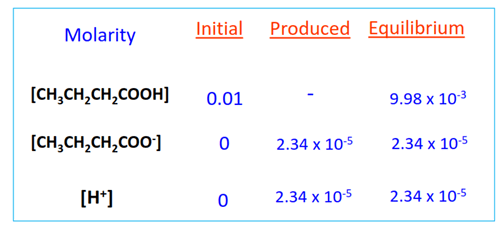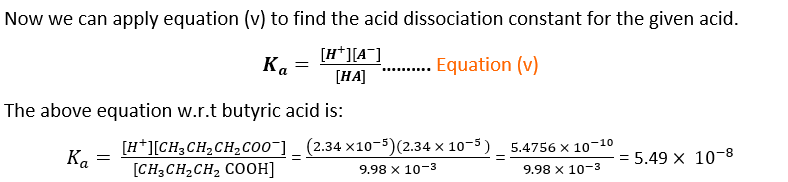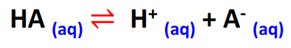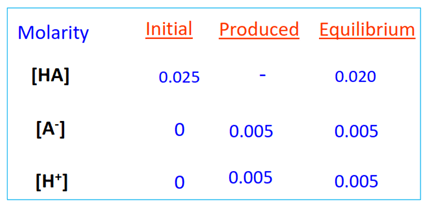# How to calculate pKa from Ka? - ka to pka, Conversion, Formulas, Equations

Home > Chemistry > How to find pKa from Ka? – (Ka to pKa)

pKa is the negative logarithm of the acid dissociation constant (Ka). It is a more convenient way of measuring the strength of an acidic solution than Ka.

You will learn in this article how to calculate the pKa value from Ka i.e. (Ka to pKa conversion) by applying a very simple but quite valuable chemical formula.

So without any further delay, dive into the article, and let’s start reading!

Page Contents

## What is pKa?

The prefix p in pKa stands for power. Just like pH determines the power of hydrogen ions present in an aqueous solution. pKa measures the strength of an acidic solution as the power of the acid dissociation constant (Ka).

pKa is calculated by taking the negative logarithm to the base 10 of the Ka value for acid, as shown in equation (i).

pKa = -log10Ka…………. Equation (i)

Weak organic acids have greater pKa values than strong mineral acids.

pKa is related to pKb i.e., base dissociation constant for an aqueous solution as shown in equation (ii).

pKa + pKb = pKw ……. Equation (ii)

Equation (ii) can be rewritten as equation (iii) by substituting the value of pKw that stays fixed at room temperature and atmospheric pressure.

pKw = water dissociation constant = 14 (at 25°C).

pKa + pKb = 14 ……. Equation (iii)

You may note that Ka measures the strength of the acid itself, while pKa is an attribute associated with the strength of the aqueous solution that the acid forms upon ionization.

## What is Ka?

Ka stands for acid dissociation constant.

The ionization equilibrium for the dissociation of a weak acid (HA) in an aqueous solution is represented as follows:The acid dissociation constant (Ka) for the above reaction can be represented as equation (iv).

$Ka = \frac{[H_{3}O^{+}][A^{-}]}{[HA][H_{2}O]}$………. Equation (iv)

Where;

• [H3O+] = concentration of hydronium ions formed in the aqueous solution
• [A] = concentration of conjugate base of the acid
• [HA] = acid concentration at equilibrium
• [H2O] = concentration of water

As water concentration stays constant throughout the reaction, while [H3O+] = [H+], i.e., the concentration of H+ ions released in the aqueous solution.

So, equation (iv) can be rearranged as equation (v).

$Ka = \frac{[H^{+}][A^{-}]}{[HA]}$………. Equation (v)

The greater the strength of an acid, the higher the Ka value for its aqueous solution and vice versa.

## What is the relationship between pKa and Ka?

This equation (pKa = -log10Ka) tells us that pKa and Ka are inversely proportional to each other. A higher Ka value results in a lower pKa value and vice versa.

This implies that a strong acid that dissociates to a large extent in an aqueous solution possesses a higher Ka value; however, it has a smaller pKa.

So pKa is also inversely related to acidic strength.## How to find pKa from Ka? – (Ka to pKa conversion)

pKa and Ka are interconvertible. The value of pKa can be easily calculated if the value of Ka is known by using equation (i) which is (pKa = -log10Ka).

We can substitute the value of Ka into equation (i) and take the negative logarithm of this value to find pKa.

pKa = -log10Ka…………. Equation (i)

It was highlighted at the beginning of the article that pKa is a more convenient way of measuring the acidity of a solution as compared to Ka.

This is because Ka for acid is usually calculated in magnitudes of 10 raised to the power x. While pKa is a small numerical value.

For instance, the Ka for acetic acid is 1.58 x 10-5; contrarily, its pKa is just 4.80.

Ka values are always positive. However, pKa values can be both positive and negative.We have provided you with the following solved examples through which you can learn how to calculate pKa from Ka i.e. (Ka to pKa conversion) by practically using the formulas given in this article.

## Solved examples of determining pKa when Ka given

 Example #1: The acid dissociation constant (Ka) value for propanoic acid (CH3CH2COOH) is 1.34 x 10-5. What is its pKa value? CH3CH2COOH is a weak acid that partially ionizes to yield H+ and CH3CH2COO– ions in water.As the Ka value for CH3CH2COOH is given in the question statement so we can find its pKa by applying the equation as shown below.∴ pKa = -log10Ka∴ pKa = -log10(1.34 x 10-5) = 4.87Result: The pKa value for propanoic acid (CH3CH2COOH) is 4.87.
 Example #2: The acid dissociation constant (Ka) value for nitric acid (HNO3) is 2.4 x 101. What is its pKa value? HNO3 is a strong acid that completely ionizes to give H+ and NO3– ions in an aqueous solution.As the Ka value for HNO3 is given in the question statement so we can find its pKa by applying the equation as shown below.∴ pKa = -log10Ka∴ pKa = -log10(2.4 x 101) = -1.38.Result: The pKa value for nitric acid (HNO3) is -1.38.A negative pKa signifies that HNO3 is an extremely strong acid. The proton is only weakly held to the acid, which it readily liberates in an aqueous solution.
 Example # 3: A chemist prepared 0.01 M butyric acid solution in his lab. How can you help the chemist find the pKa of the acidic solution if its pH at the equilibrium point is reported in the literature as pH= 4.63? Butyric acid, also known as butanoic acid (CH3CH2CH2COOH), is a weak acid that partially dissociates to release H+ and butanoate (CH3CH2CH2COO–) ions in an aqueous solution.As the pH of the solution is given in the question statement, so we can find its [H+] at equilibrium using equation (vi).∴ [H+]equilibrium = 10-pH ………. Equation (vi)∴ [H+]equilibrium = 10-4.63 = 2.34 x 10-5 M.As per the balanced chemical equation shown above;⇒ [H+]equilibrium = [CH3CH2CH2COO–]equilibrium So [CH3CH2CH2COO–]equilibrium = [H+]equilibrium = 2.34 x 10-5 M.The initial butyric acid concentration is also given in the question, so[CH3CH2CH2COOH]equilibrium = [CH3CH2CH2COOH]initial – [CH3CH2CH2COO–]equilibrium= 0.01 – (2.34 x 10-5) = 9.98 x 10-3 M.Now that we know the acid dissociation constant (Ka) value, we can easily find pKa by applying equation (i).∴ pKa = -log10Ka…………. Equation (i)∴ pKa = -log10(5.49 x 10-8) = 7.26Result: The pKa value for the butyric acid solution is 7.26.A comparatively high pKa denotes a low acidic strength.
 Example # 4: The Ka value for a weak acid is given to be 2.33 x 10-11. Which of the following options provides the correct pKa value for its acidic solution?A) 11.23            B) 10.93           C) 10.63         D) 11.83        E) 9.93 Answer: Option C (pKa = 10.63) is the correct answer.Explanation: pKa can be determined from the already given Ka value by applying equation (i).pKa = -log10Ka …………. Equation (i)pKa = -log10(2.33 x 10-11) = 10.63
 Example # 5: A 0.025 M solution of a weak acid dissociates to produce 0.005 mol/L hydrogen ions at the equilibrium stage. Find its pKa. A weak acid (HA) partially dissociates to liberate H+ and A– ions in water.The concentration of hydrogen ions released at equilibrium is given in the question statement i.e., [H+] equilibrium = 0.005 M.∴ [H+] equilibrium = [A–]equilibrium = 0.005 MThe original concentration of HA is also given in the question statement, so [HA]equilibrium = [HA]initial – [H+] equilibrium = 0.025 – 0.005 = 0.02 M.As a final step, we just need to substitute the above value into equation (i) to find the required pKa value.∴ pKa = -log10Ka …………. Equation (i)∴ pKa = -log10(1.25 x 10-3) = 2.90Result: The pKa value for the given acidic solution is 2.90.

Also check:

## FAQ

### What is Ka?

Ka stands for acid dissociation constant.

A weak acid (HA) partially dissociates to produce H+ and A ions in water. H+ ions combine with H2O molecules to form hydronium (H3O+) ions. A is known as the conjugate base of the acid. HA and A together are known as a conjugate acid-base pair.

The equilibrium constant for a reversible reaction is the ratio of the product of the concentration of products to the product of reactant concentrations.The ionization equilibrium for the dissociation of HA in an aqueous solution can be represented as follows:

⇒ HA + H2O ⇌ H3O+ + A

The equilibrium constant (Ka) for the above reaction can be represented as equation (i)

$Ka = \frac{[H_{3}O^{+}][A^{-}]}{[HA][H_{2}O]}$………. Equation (i)

Where;

• [H3O+] = concentration of hydronium ions formed in the aqueous solution
• [A] = concentration of conjugate base of the acid
• [HA] = acid concentration at equilibrium
• [H2O] = concentration of water

As water concentration stays constant throughout the reaction, while [H3O+] = [H+], i.e., the concentration of H+ ions released in the aqueous solution. So, equation (i) can be rearranged as equation (ii).

$Ka = \frac{[H^{+}][A^{-}]}{[HA]}$………. Equation (ii)

The greater the strength of an acid, it undergoes dissociation to a larger extent in the aqueous solution; thus, it possesses a higher Ka value.

Weak organic acids such as acetic acid and citric acid have Ka values below 1. However, strong mineral acids such as HCl and HNO3 that completely dissociates to release a large number of H+ ions in an aqueous solution have Ka values above 1.

### What is pKa?

pKa measures the strength of an acidic solution. It is the negative logarithm of the Ka value for an acid.

### How is pKa related to the strength of a Bronsted acid?

As per the Bronsted-Lowry acid-base theory, an acid is defined as a proton donor. The proton is loosely held to a strong acid which can readily liberate it in an aqueous solution.

However, weak acids strongly hold their protons, not ready to release in water.

Greater the pKa value, the more strongly held the proton is by the Bronsted acid indicating a weak acidic strength and vice versa.

So pKa is inversely related to the strength of a Bronsted acid.### How is Ka related to the strength of an acid?

Higher the Ka value, the greater the strength of an acid. So the acid dissociation constant is directly related to the strength of an acid.Strong acids completely ionize in water to release H+ ions. Thus, they have high Ka values.

Weak acids only partially ionize in water to release a limited number of H+ ions, so they have low Ka values.

### What is the relationship between pKa and Ka?

pKa is inversely related to Ka. The higher the Ka value of an acid, the lower its pKa and vice versa.

### What is the formula to calculate pKa from Ka?

pKa can be calculated by taking the negative logarithm of Ka as follows;

∴ pKa = -log10Ka.

### How do you calculate Ka from pKa?

Ka and pKa are interconvertible chemical parameters. Ka can be calculated from pKa by taking the antilog of pKa as follows;

∴ Ka = 10-pKa

## Summary

• pKa stands for the power of Ka. It measures the acidic strength of an aqueous solution.
• It is calculated as a negative logarithm of Ka to the base 10.
• pKa = -log10Ka.
• The greater the strength of an acid, the lower its pKa value, and vice versa.
• Ka denotes acid dissociation constant. It measures the extent of ionization of an acid in an aqueous solution.
• Greater the Ka value, the higher the strength of the acid.
• If the value of Ka for acid is known, we can easily find its pKa value by taking the negative logarithm of Ka. The formula to determine pKa from Ka is [pKa = -log10Ka].
Did you like it?

#### Ammara Waheed

Ammara Waheed is a highly qualified and experienced chemist, whose passion for Chemistry is evident in her writing. With a Bachelor of Science (Hons.) and Master of Philosophy (M. Phil) in Physical and Analytical Chemistry from Government College University (GCU) Lahore, Pakistan, with a hands-on laboratory experience in the Pakistan Council of Scientific and Industrial Research (PCSIR), Ammara has a solid educational foundation in her field. She comes from a distinguished research background and she documents her research endeavors for reputable journals such as Wiley and Elsevier. Her deep knowledge and expertise in the field of Chemistry make her a trusted and reliable authority in her profession. Let's connect - https://www.researchgate.net/profile/Ammara-Waheed

Share it...